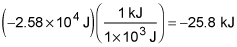##### Physics I: 501 Practice Problems For Dummies (+ Free Online Practice)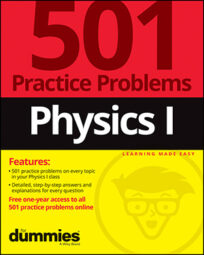When you push an object on a slope, you can use physics to calculate the amount of force that is applied. For example, you can calculate how much force someone applies to push a sled down a snow-covered hill.

Here are some practice questions that you can try.

## Practice questions

Use the following information to answer the questions: A father pushes a sled carrying his child down a 200-meter-high hill with a 32-degree slope. Using a constant force of 500 newtons directed parallel to the hill's surface, he increases the sled's velocity from 0 to 4.2 meters per second in 5 seconds, at which point he lets go. The combined mass of the child and the sled is 65 kilograms.

1. How much work does the father do pushing his child?

2. How many kilojoules of work does friction do between the time that the father lets go of the sled and the time that the sled reaches the bottom of the hill?

The coefficient of kinetic friction between the sled's runners and the snow is 0.13. Round your answer to the nearest integer.

The following are the answers to the practice questions:

1. 5,250 J

Use the work formula,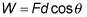where W is the amount of work done on an object, F is the magnitude of the force exerted on the object, d is the distance the object moves, and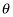is the angle between the force and distance vectors. Because the 500-newton force is parallel to the hill's surface, it must be in the same direction as the distance the sled travels while it's being pushed. In that case,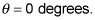To solve for the distance the sled travels, you need to know the sled's acceleration given the initial and final velocities, and you need to know the time. Start with the velocity-time formula: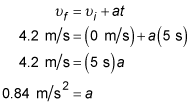Follow up by using the displacement formula or the velocity-displacement formula (used here) to solve for the displacement/distance traveled: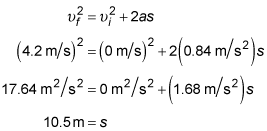Finally, substitute this into the work formula along with the information given in the problem setup.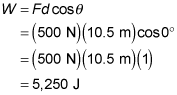2. –26 kJ

First you need to find out how far the sled travels.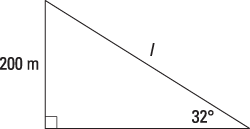Use trigonometry to calculate the entire length of the hill's surface: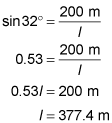You can determine how far the sled travels while the father pushes it by first finding the acceleration with the velocity-time formula: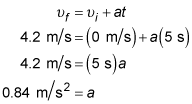Then use the displacement formula or the velocity-displacement formula (used here) to solve for the displacement: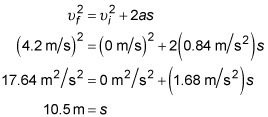That leaves 377.4 m – 10.5 m = 366.9 m for the no-push portion of the sled's descent. Then, to find the amount of force that friction exerts after the pushing stops, draw a free-body diagram: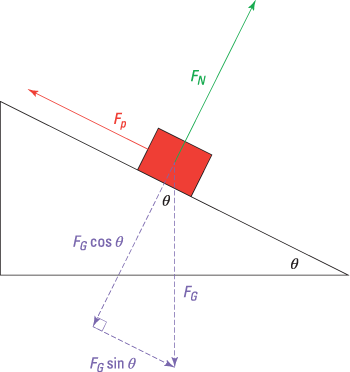Use Newton's second law in the vertical direction (FG = maG = mg on the surface of Earth):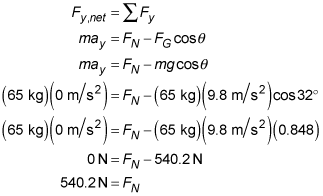Use the definition of friction,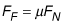to solve for the force of friction.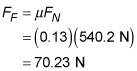Then use that value in the work formula. Because the force of friction runs opposite to the motion of an object, the angle between the force and distance vectors is 180 degrees.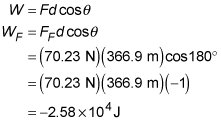Finally, convert into the desired units: